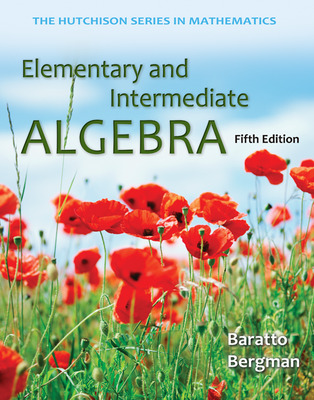# Loose Leaf Version for Elementary and Intermediate Algebra

5th Edition
By Stefan Baratto and Barry Bergman and Donald Hutchison
ISBN10: 0077574494
ISBN13: 9780077574499

## Purchase Options

Students, we’re committed to providing you with high-value course solutions backed by great service and a team that cares about your success. See tabs below to explore options and pricing. Don't forget, we accept financial aid and scholarship funds in the form of credit or debit cards.

### Product

The estimated amount of time this product will be on the market is based on a number of factors, including faculty input to instructional design and the prior revision cycle and updates to academic research-which typically results in a revision cycle ranging from every two to four years for this product. Pricing subject to change at any time.

## Preface Applications Index

### Chapter 0. Prealgebra Review

0.1 A Review of Fractions

0.2 Real Numbers

0.4 Multiplying and Dividing

0.5 Exponents and Order of Operations

Chapter 0: Summary

Chapter 0: Summary Exercises

Chapter 0: Chapter Test

### Chapter 1. From Arithmetic to Algebra

1.1 Transition to Algebra

Activity 1: Exchanging Money

1.2 Evaluating Algebraic Expressions

1.3 Simplifying Algebraic Expressions

1.4 Solving Equations with the Addition Property

1.5 Solving Equations with the Multiplication Property

1.6 Combining the Rules to Solve Equations

1.7 Linear Inequalities

Chapter 1: Summary

Chapter 1: Summary Exercises

Chapter 1: Chapter Test

### Chapter 2. Functions and Graphs

2.1 Formulas and Problem Solving

Activity 2: Graphing with a Calculator

2.2 Sets and Set Notation

2.3 Two Variable Equations

2.4 The Cartesian Coordinate System

2.5 Relations and Functions

2.6 Tables and Graphs

Chapter 2: Summary

Chapter 2: Summary Exercises

Chapter 2: Chapter Test

Chapters 0-2: Cumulative Review

### Chapter 3. Graphing Linear Functions

3.1 Graphing Linear Functions

Activity 3: Linear Regression: A Graphing Calculator Activity

3.2 The Slope of a Line

3.3 Linear Equations

3.4 Rate of Change and Linear Regression

3.5 Linear Inequalities in Two Variables

Chapter 3: Summary

Chapter 3: Summary Exercises

Chapter 3: Chapter Test

Chapters 0-3: Cumulative Review

### 4. Systems of Linear Equations

4.1 Graphing Systems of Linear Equations

Activity 4: Agricultural Technology

4.2 Solving Equations in One Variable Graphically

4.3 Systems of Equations in Two Variables

4.4 Systems of Equations in Three Variables

4.5 Systems of Linear Inequalities

Chapter 4: Summary

Chapter 4: Summary Exercises

Chapter 4: Chapter Test

Chapters 0-4: Cumulative Review

### Chapter 5. Exponents and Polynomials

5.1 Positive Integer Exponents

Activity 5: Wealth and Compound Interest

5.2 Integer Exponents and Scientific Notation

5.3 Introduction to Polynomials

5.5 Multiplying Polynomials and Special Products

5.6 Dividing Polynomials

Chapter 5: Summary

Chapter 5: Summary Exercises

Chapter 5: Chapter Test

Chapters 0-5: Cumulative Review

### Chapter 6. Factoring Polynomials

6.1 An Introduction to Factoring

Activity 6: ISBN’s and the Check Digit

6.2 Factoring Special Polynomials

6.3 Factoring: Trial and Error

6.4 Factoring: The ac Method

6.5 Factoring Strategies

6.6 Factoring and Problem Solving

Chapter 6: Summary

Chapter 6: Summary Exercises

Chapter 6: Chapter Test

Chapters 0-6: Cumulative Review

### Chapter 7. Radicals and Exponents

Activity 7: The Swing of a Pendulum

7.5 Rational Exponents

7.6 Complex Numbers

Chapter 7: Summary

Chapter 7: Summary Exercises

Chapter 7: Chapter Test

Chapters 0-7: Cumulative Review

Activity 8: The Gravity Model

8.3 An Introduction to Parabolas

8.4 Quadratic Equations and Problem Solving

Chapter 8: Summary

Chapter 8: Summary Exercises

Chapter 8: Chapter Test

Chapters 0-8: Cumulative Review

### Chapter 9. Rational Expressions

9.1 Simplifying Rational Expressions

Activity 9: Communicating Mathematical Ideas

9.2 Multiplying and Dividing Rational Expressions

9.3 Adding and Subtracting Rational Expressions

9.4 Complex Rational Expressions

9.5 Graphing Rational Functions

9.6 Rational Equations and Problem Solving

Chapter 9: Summary

Chapter 9: Summary Exercises

Chapter 9: Chapter Test

Chapters 0-9: Cumulative Review

### Chapter 10. Exponential and Logarithmic Functions

10.1 Algebra of Functions

Activity 10: Half-Life and Decay

10.2 Composition of Functions

10.3 Inverse Functions

10.4 Exponential Functions

10.5 Logarithmic Functions

10.6 Properties of Logarithms

10.7 Logarithmic and Exponential Equations

Chapter 10: Summary

Chapter 10: Summary Exercises

Chapter 10: Chapter Test

Chapters 0-10: Cumulative Review

### Appendices

A.1 Solving Inequalities in One Variable Graphically

A.2 Solving Absolute-Value Equations

A.3 Solving Absolute-Value Equations Graphically

A.4 Solving Absolute-Value Inequalities

A.5 Solving Absolute-Value Inequalities Graphically